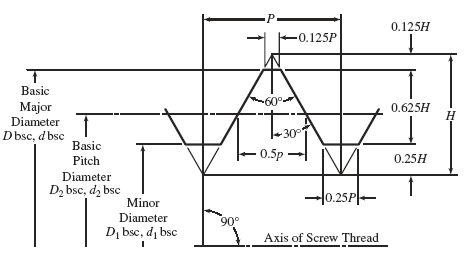Related Resources: calculators

### UN External Screw Threads Formulas and Calculator

Inch Size UN External Screw Threads Formulas and Calculator per. ASME B1.1

The formulas below are for inch screw threads are based on those listed in ASME B1.1, Unified Inch Screw Threads.

Screw Threads Calculator for Internal Unified Inch ScrewFormulas Used:
Note that calculated values may be slightly different than table reference data due to math rounding differences.

Maximum external major diameter

Eq. 1
dmax. = dbsc − es

Eq. 2

where

dbsc = Basic major diameter
es = Allowance

• Classes 1A and 2A, es = 0.300 & Class 2A pitch diameter tolerance
•  Class 3A, es = no allowance

Major Diameter Tolerance

(1 ) Class 1A = 0.090 P2/3
(2) Classes 2A and 3A = 0.060 P2/3
The tolerance for Class 2A coarse and the 8-thread series threads of unfinished, hot-rolled material is0.0903P2/3.
This does not apply to standard fasteners with rolled threads.

Minimum dia major diameter
Eq. 3
dmin. = dmax. − Td

where

Class 1A Td = 0.090 P2/3
Classes 2A and 3A Td = 0.060 P2/3
The tolerance for Class 2A coarse and the 8-thread series threads of unfinished, hot-rolled material is0.0903P2/3. This does not apply to standard fasteners with rolled threads.

Maximum external pitch diameter
Eq. 4
d2max. = dmax. − 2has

where

dmax = Maximum external major diameter
2has = 0.64951905P

Minimum external pitch diameter
Eq. 5
d2min. = d2max. − Td2

where

d2 = 0.0015 (d bsc)1/3 + 0.0015 LE1/2 0.015 (P2)1/3

Maximum external UNR minor diameter

Eq. 6
d3max. = dmax. − 2hs

where

2hs = 1 .19078493P
dmax. = Maximum external major diameter

Maximum external UN minor diameter

Eq. 7
d1max. = dmax. − 2hs

where

2hs = 1 .08253175P
dmax. = Maximum external major diameter

LE = length of engagement
P = thread pitch = 1/n
n = number of threads per inch

Notes

(a) Maximum Major Diameter (External Threads)
(1 ) For Classes 1A and 2A, d = basic major diameter, Dbsc, minus allowance.
(2) For Class 3A, d = basic major diameter, Dbsc.

(b) Minimum Major Diameter (External Threads). For all classes, equals maximum major diameter, minus major diameter tolerance for respective class of thread.

(c) Maximum Pitch Diameter (External Threads)
(1 ) For Classes 1A and 2A, d2 =basic pitch diameter D2bsc, minus allowance.
(2) For Class 3A, d2 = basic pitch diameter D2bsc.

(d) Minimum Pitch Diameter (External Threads). For all classes, equals maximum pitch diameter, minus pitch diameter tolerance for respective class of thread.

(e) Maximum Minor Diameter (External Threads). In dimensioning UN series external threads, the minor diameter is not specified. In practice, the minor diameter is satisfactory when accepted by a standard GO thread gage in accordance with ASME B1.2. When it is desirable to obtain minor diameter values for reference purposes and for UNR threads, they can be calculated as follows:

(1 ) For UN series threads, the formulas are:
(a) for Classes 1A and 2A, d1 = basic minor diameter D1bsc, minus allowance
(b) for Class 3A, d1 = basic minor diameter D1bsc
(2) For UNR series threads, the formulas are:
(a) for Classes 1A and 2A, d3 p basic minor diameter D1bsc, minus allowance, minus H/8
(b) or Class 3A, d3 = basic minor diameter D1bsc, minus H/8

(f) Minimum Minor Diameter (External Threads). When it is desirable for design purposes to calculate the minimum diameter, it can be obtained for all classes by the formula: minimum pitch diameter minus 0.64951905P.

Related: